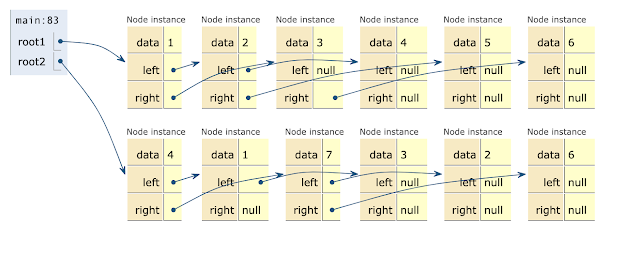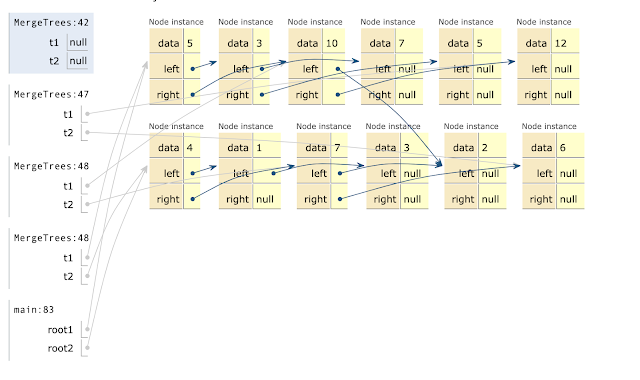## Wednesday, May 18, 2022

### Easy_Question19 : Merge Two Binary Trees

• You are given two binary trees `root1` and `root2`.
• Imagine that when you put one of them to cover the other, some nodes of the two trees are overlapped while the others are not. You need to merge the two trees into a new binary tree. The merge rule is that if two nodes overlap, then sum node values up as the new value of the merged node. Otherwise, the NOT null node will be used as the node of the new tree.
• Return the merged tree.
• Note: The merging process must start from the root nodes of both trees.

`Example:  Input:      Tree 1            Tree 2                         2                 3                                   / \               / \                                 1   4             6   1                            /                   \   \                         5                     2   7                  Output: Merged tree:         5        / \       7   5      / \     \     5   2   7 `
```Recursive Algorithm: Traverse the tree in Pre-order fashionCheck if both the tree nodes are NULL If not, then update the valueRecur for left subtreesRecur for right subtreesReturn root of updated Tree// Java program to Merge Two Binary Trees/* A binary tree node has data, pointer to left childand a pointer to right child */class Node{  int data;  Node left, right;    public Node(int data, Node left, Node right) {    this.data = data;    this.left = left;    this.right = right;  }    /* Helper method that allocates a new node with the  given data and NULL left and right pointers. */  static Node newNode(int data)  {    return new Node(data, null, null);  }    /* Given a binary tree, print its nodes in inorder*/  static void inorder(Node node)  {    if (node == null)      return;      /* first recur on left child */    inorder(node.left);      /* then print the data of node */    System.out.printf("%d ", node.data);      /* now recur on right child */    inorder(node.right);  }    /* Method to merge given two binary trees*/  static Node MergeTrees(Node t1, Node t2)  {    if (t1 == null)      return t2;    if (t2 == null)      return t1;    t1.data += t2.data;    //Step 2 start     t1.left = MergeTrees(t1.left, t2.left);    //Step 2 end     //Step 3 start     t1.right = MergeTrees(t1.right, t2.right);    //Step 3 end     return t1;  }    // Driver method  public static void main(String[] args)  {    /* Let us construct the first Binary Tree        1      / \      2  3      / \  \      4 5  6    */    //Step 1 start    Node root1 = newNode(1);    root1.left = newNode(2);    root1.right = newNode(3);    root1.left.left = newNode(4);    root1.left.right = newNode(5);    root1.right.right = newNode(6);      /* Let us construct the second Binary Tree        4      / \      1  7      /  / \    3  2 6 */    Node root2 = newNode(4);    root2.left = newNode(1);    root2.right = newNode(7);    root2.left.left = newNode(3);    root2.right.left = newNode(2);    root2.right.right = newNode(6);    //Step 1  end    Node root3 = MergeTrees(root1, root2);    System.out.printf("The Merged Binary Tree is:\n");    //Step 4    inorder(root3);  }}Output: The Merged Binary Tree is:
7 3 5 5 2 10 12Step1 VisualizerStep2 Left Tree merge VisualizerStep3 Rigth Tree merge VisualizerStep4 Merged Tree VisualizerComplexity Analysis: Time complexity : O(n) A total of n nodes need to be traversed. Here, n represents the minimum number of nodes from the two given trees.Auxiliary Space : O(n) The depth of the recursion tree can go upto n in case of a skewed tree. In average case, depth will be O(logn).```

## You may also like

a
Kubernetes AWS Java Coding Question
Microservices Core Java Python
Spring Framework AI/MLSpring Boot